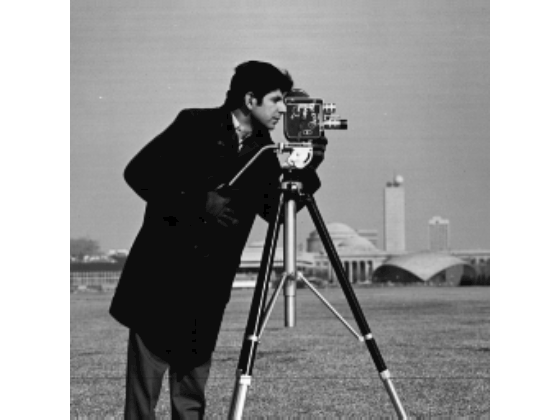# denoiseImage

Denoise image using deep neural network

## Syntax

``B = denoiseImage(A,net)``

## Description

example

````B = denoiseImage(A,net)` estimates denoised image `B` from noisy image `A` using a denoising deep neural network specified by `net`.This function requires that you have Deep Learning Toolbox™.```

## Examples

collapse all

Load the pretrained denoising convolutional neural network, `'DnCNN'`.

`net = denoisingNetwork('DnCNN');`

Load a grayscale image into the workspace, then create a noisy version of the image.

```I = imread('cameraman.tif'); noisyI = imnoise(I,'gaussian',0,0.01);```

Display the two images as a montage.

```montage({I,noisyI}) title('Original Image (Left) and Noisy Image (Right)')```Remove noise from the noisy image, then display the result.

```denoisedI = denoiseImage(noisyI,net); imshow(denoisedI) title('Denoised Image')```## Input Arguments

collapse all

Noisy image, specified as a single 2-D image or a stack of 2-D images. `A` can be:

• A 2-D grayscale image with size m-by-n.

• A 2-D multichannel image with size m-by-n-by-c, where c is the number of image channels. For example, c is 3 for RGB images, and 4 for four-channel images such as RGB images with an infrared channel.

• A stack of equally-sized 2-D images. In this case, `A` has size m-by-n-by-c-by-p, where p is the number of images in the stack.

Data Types: `single` | `double` | `uint8` | `uint16`

Denoising deep neural network, specified as a `SeriesNetwork` (Deep Learning Toolbox) object. The network should be trained to handle images with the same channel format as `A`.

## Output Arguments

collapse all

Denoised image, returned as a single 2-D image or a stack of 2-D images. `B` has the same size and data type as `A`.